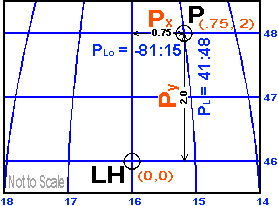## L,Lo to XY Conversion || Dan's Home Page | Pyxis Home Page | Map Page ||

Converting lattitude and longitude to XY coordinates for the maps I make is a fairly straight forward process involving just a little math. For my maps of the Fairport Harbor area I use the Fairport Harbor lighthouse as an origin (x,y = 0,0). Lat & long are converted to the x,y distance from the lighthouse in nautical miles. The lattitude is the y distance and the longitude is the x distance. The y distance is pretty simple. It is the difference in minutes of lattitude. 1 minute of lattitude = 1 natical mile. If the point is 1 minute north of the light house the y coordinate is 1.00. The longitude conversion is complicated a bit by the fact that the earth is round. But for the large scale (small area) maps I make it is pretty simple. Since the lines of longitude converge as you move north 1 minute of longitude = 1 nautical mile only at the equator. At the pole 1 minute of longitude = 0 nautical miles. The distance varies with the cosine of the lattitude so all we have to do is multiply the difference in longitude by the cosine of the lattitude. Here are the formulas:X = DLo * cos(L)
Y = DL

DL is the difference in lattitude in minutes
DLo is the difference in longitude in minutes
L is the lattitude of the point being converted.
DL and Dlo are measured relative to the light house.
For these examples I am taking west longitude to be negative.

Example:
Light House (LH)
L = 41:46
Lo = -81:16

Point P
L = 41:48
Lo = -81:15

X = (Lo(P) - Lo(LH)) * cos(L(P))
X = ((-81:16) - (-81:15)) * cos(41:46)
X = (1.0) * (0.75)
X = 0.75

Y = L(P) - L(LH)
Y = (41:48) - (41:46)
Y = 2.00

The XY coordinates of the point P relative to the light house LH are .75, 2.0. The conversion is an approximation. At the scales I am working with for these maps the difference is not significant. It is even less significant when you consider the pixel size in the maps. On the map of Fairpot Harbor each pixel represents about 20 feet. The error from treating this small area as if it were flat after the lat, long to x,y conversion is on the order of inches.

Some References on Coordinate Conversion

The American Practical Navigator (Bowditch)
Defense Mapping Agency Hydrographic/Topographic Center
Bethesda, Maryland 1995

Dent, Borden
Cartography, Thematic Map Design
Georgia State University 1990

Keys, Gerry
Practical Navigation by Calculator
Stanford Maritime
London, England 1984

Maling, D.H.
Coordinate Systems and Map Projections
Pergamon Press
Oxford, England 1992